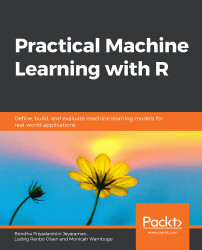•#### Practical Machine Learning with R#### Overview of this book

With huge amounts of data being generated every moment, businesses need applications that apply complex mathematical calculations to data repeatedly and at speed. With machine learning techniques and R, you can easily develop these kinds of applications in an efficient way. Practical Machine Learning with R begins by helping you grasp the basics of machine learning methods, while also highlighting how and why they work. You will understand how to get these algorithms to work in practice, rather than focusing on mathematical derivations. As you progress from one chapter to another, you will gain hands-on experience of building a machine learning solution in R. Next, using R packages such as rpart, random forest, and multiple imputation by chained equations (MICE), you will learn to implement algorithms including neural net classifier, decision trees, and linear and non-linear regression. As you progress through the book, you’ll delve into various machine learning techniques for both supervised and unsupervised learning approaches. In addition to this, you’ll gain insights into partitioning the datasets and mechanisms to evaluate the results from each model and be able to compare them. By the end of this book, you will have gained expertise in solving your business problems, starting by forming a good problem statement, selecting the most appropriate model to solve your problem, and then ensuring that you do not overtrain it.Free Chapter
An Introduction to Machine LearningData Cleaning and Pre-processingFeature EngineeringIntroduction to neuralnet and Evaluation MethodsLinear and Logistic Regression ModelsUnsupervised LearningAppendix## k-means Clustering

The k-means clustering algorithm is one of the most popular clustering techniques. It produces hard (an element can only be a member of one cluster), flat, and polythetic (membership is determined by similarity based on multiple attributes) clusters. The k-means algorithm has no training or testing data per se. It works by creating clusters around centroids. A centroid is an average cluster member; that is, the center of a cluster. k-means requires us to specify the number of clusters (k). It is important to note that the number of clusters specified greatly affects the performance of the k-means algorithm. Deciding on the number of clusters can be informed by domain knowledge. For example, knowing about the features of a given dataset will help to set parameters for clusters. In situations where this information is not available, there are two techniques we can use to help us decide on the correct number of clusters.

In situations...